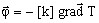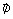# Thermal conductivity: definitions

## Definition

The thermal conductivity k is a thermo-physical material property, which characterizes the quantity of heat transferred through a unit surface area in a time unit when the heat transfer is generated by a temperature gradient.

This quantity appears in Fourier's law:where:

•is the flux density of the heat (W/m2)
• k is the tensor of the thermal conductivity (W/m/degree)
• T is the temperature (in degrees)

The thermal conductivity characterizes the ability of the material to transfer heat.

## Dependence on temperature

The thermal conductivity can depend on the temperature: k=f(T). It increases or decreases when the temperature increases.